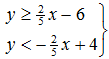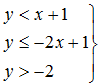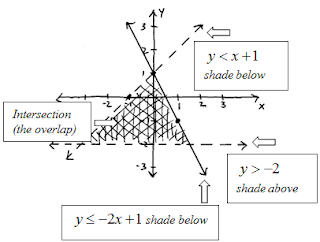## Pages

### Systems of Linear Inequalities (Two Variables)

The systems of linear inequalities that we will be solving consist of two linear inequalities and two variables.  To solve these we will graph the solution sets of both linear inequalities and then determine where the sets intersect.  Any point in the overlap of the graphs will be a solution to the system.

Instructional Video: Systems of Linear Inequalities

Graph the solution set:The above solution suggests that (−5, 3) is a solution because it is shaded.  Check it and others to see if it solves both inequalities.These graphs can sometimes get messy so do your best to think about the solution before actually shading.  Use pencil and a good eraser when working on these problems.

Given the graph, determine the system.Graph the systems of inequalities.Graph the system: